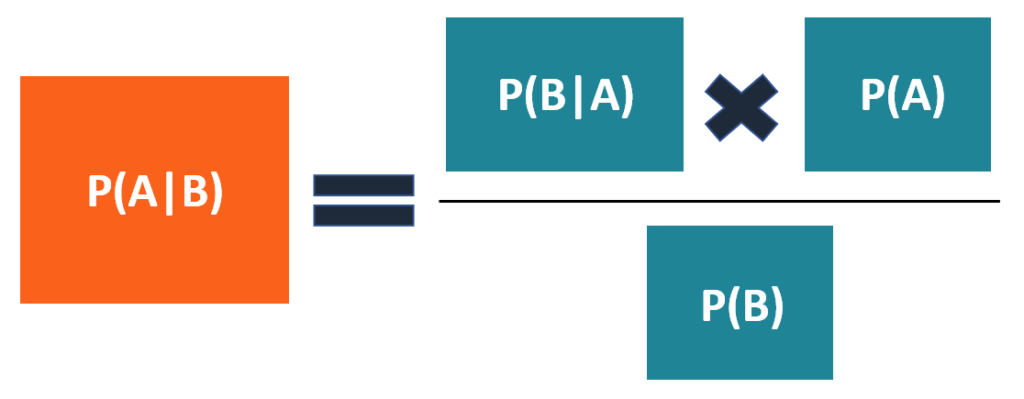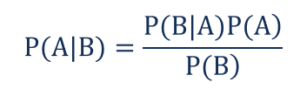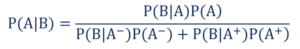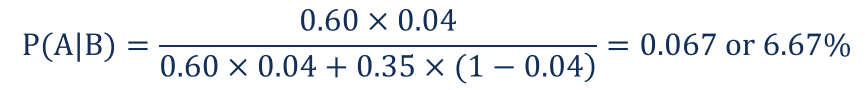# Bayes’ Theorem

A mathematical formula used to determine the conditional probability of events

## What is the Bayes’ Theorem?

In statistics and probability theory, the Bayes’ theorem (also known as the Bayes’ rule) is a mathematical formula used to determine the conditional probability of events. Essentially, the Bayes’ theorem describes the probability of an event based on prior knowledge of the conditions that might be relevant to the event.

The theorem is named after English statistician Thomas Bayes, who discovered the formula in 1763. It is considered the foundation of the special statistical inference approach called the Bayes’ inference.Besides statistics, the Bayes’ theorem is also used in various disciplines, with medicine and pharmacology as the most notable examples. In addition, the theorem is commonly employed in different fields of finance. Some of the applications include, but not limited to, modeling the risk of lending money to borrowers or forecasting the probability of the success of an investment.

### Formula for Bayes’ Theorem

The Bayes’ theorem is expressed in the following formula:Where:

• P(A|B) – the probability of event A occurring, given event B has occurred
• P(B|A) – the probability of event B occurring, given event A has occurred
• P(A) – the probability of event A
• P(B) – the probability of event B

Note that events A and B are independent events (i.e., the probability of the outcome of event A does not depend on the probability of the outcome of event B).

A special case of the Bayes’ theorem is when the event A is a binary variable. In such a case, the theorem is expressed in the following way:Where:

• P(B|A) – the probability of event B occurring given that event A has occurred
• P(B|A+) – the probability of event B occurring given that event A+ has occurred

In the special case above, events A and A+ are mutually exclusive outcomes of event A.

### Example of Bayes’ Theorem

Imagine you are a financial analyst at an investment bank. According to your research of publicly-traded companies, 60% of the companies that increased their share price by more than 5% in the last three years replaced their CEOs during the period.

At the same time, only 35% of the companies that did not increase their share price by more than 5% in the same period replaced their CEOs. Knowing that the probability that the stock prices grow by more than 5% is 4%, find the probability that the shares of a company that fires its CEO will increase by more than 5%.

Before finding the probabilities, you must first define the notation of the probabilities.

• P(A) – the probability that the stock price increases by 5%
• P(B) – the probability that the CEO is replaced
• P(A|B) – the probability of the stock price increases by 5% given that the CEO has been replaced
• P(B|A) – the probability of the CEO replacement given the stock price has increased by 5%.

Using the Bayes’ theorem, we can find the required probability:Thus, the probability that the shares of a company that replaces the CEO will grow by more than 5% is 6.67%.